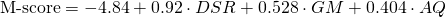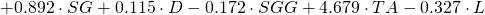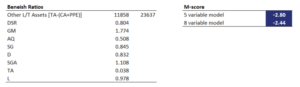# Beneish M-score

The Beneish M-score is a finance model proposed by Messod Beneish in 1997. The model can help analysts to detect financial fraud. The M-score model tries to determine the likelihood that the management of a company is manipulating the balance sheet and/or the income statement. There are two versions of the M-score model, the 5 variable model and the 8 variable model. Here we discuss the 8-variable model, which is the most widely used version of Beneish’s model.

On this page, we discuss the M-score, why the M-score model may have stopped working in recent years, and provide a numerical example. A Beneish M-score calculator is available in Excel at the bottom of the page. In the Excel calculator, we implement both the 5-variable model and the 8-variable model.

## Beneish M-score definition

To calculate the M-score, we first need to calculate a set of financial ratios. These are sometimes referred to as the ‘Beneish ratios’. The ratios capture changes in key financial variables using data from both the balance sheet and the income statement. The Beneish ratios we need to look at are the following

• Days Sales in Receivables (DSR)
• Gross Margin (GM)
• Asset Quality (AQ)
• Sales Growth (SG)
• Depreciation (D)
• Sales, General, and administrative expenses (SGG)
• Leverage (L)
• total accruals (TA)

## Beneish M-score formula

Once we have calculated all the above ratios, we then need to fill in the values in the following formula:The resulting M-score should be interpreted as follows. If the M-score is less than -2.22, then this means that the company is unlikely to be manipulating the statements. If the M-score is larger than -2.22, then it means that the company is probably manipulating the financial statements.

There are three main disadvantages to using the M-score model. First, since managers know that analysts are using this specific model to detect manipulation, managers will make sure that they stay undetected. Thus, management is unlikely to manipulate entries of the balance sheet that are included M-score model. Thus, the M-score model can be expected to work less well as the management of a company learns about the model.

The second disadvantage is that the M-model is a probabilistic model. This means that it cannot detect companies that manipulate their financial statements. Instead, it can only give the user a probability.

Finally, the model cannot be applied to financial firms, because they were not included by Messod Beneish when he estimated the model.

## Comparison Beneish M-score versus Altman z-score

The Beneish M-score is similar to the Altman z-score. Unlike the Altman z-score, however, the M-score tries to estimate the probability that a company is cooking the books. Altman’s z-score is used to estimate the probability that a company will go bankrupt.

## M-score model example

Let’s have a look at a concrete example. Suppose we calculate the following Beneish ratios. Then we use the above equation to calculate the M-score:In this case, with a score of -2.44, the company is probably a manipulator. The analyst should therefore be cautious. The Beneish M-score calculator in the screenshot is available at the bottom of the page.

### M-score calculator

Want to have an implementation in Excel? Download the Excel file: Beneish M-score